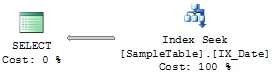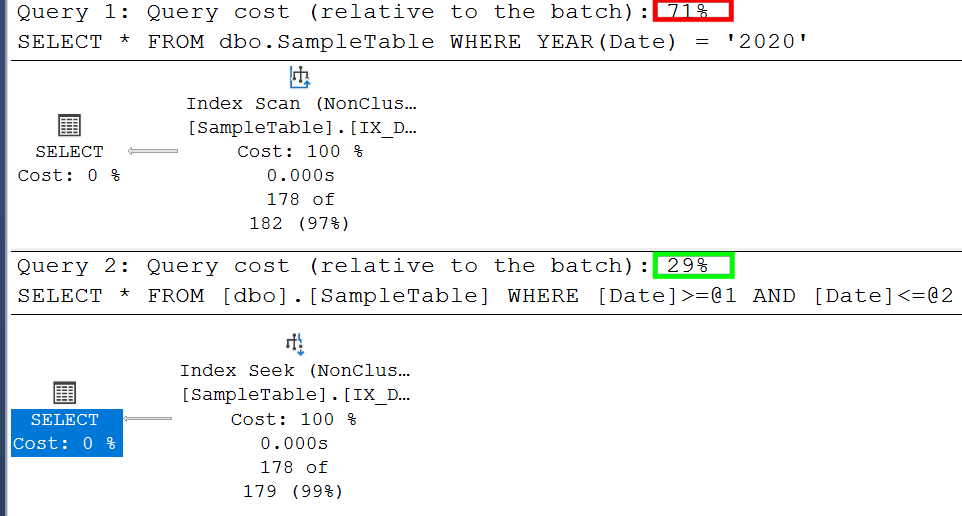SQL Server

# Why to avoid functions in WHERE?

It’s well known that we should use functions in the WHERE clause because they can have a negative impact or query execution because of the suboptimal execution plan is generated. I have prepared a really simple example of how to demonstrate this. It also demonstrates one rule that we should follow as much as possible when writing queries: never try to cast column value in comparison if there is any other option on how to evaluate it against scalar expression.

Let’s see our example. We will create table [dbo].[SampleTable] with [Date] column and populate it with some sample data. Then we will create an index over the [Date] column which one we will expect to be used later by sample query.

```-- Create sample table
CREATE TABLE [dbo].[SampleTable]
(
[Id] INT IDENTITY(1,1) PRIMARY KEY,
[Date] DATETIME
)
GO

-- Populate table with sample data
INSERT [dbo].[SampleTable]
SELECT TOP 2000 DATEADD(dd, ROW_NUMBER() OVER (ORDER BY [o1].[object_Id], [o2].[object_Id]),
FROM [sys].[objects] [o1]
CROSS JOIN [sys].[objects] [o2]
GO

-- Create index on [Date] column
CREATE NONCLUSTERED INDEX [IX_Date] ON [dbo].[SampleTable]
(
[Date] ASC
)
GO```

We will execute the following query to get all data for the specified year and check the execution plan:

`SELECT * FROM [dbo].[SampleTable] WHERE YEAR([Date]) = '2011'`Index Scan was used to get requested data. This is not exactly what we would like to see because we have created an index and based od data selectivity we know that only a small subset of rows from the table should be returned. It is caused by the fact that we have used YEAR() function over the [Date] column which prevents the use of index seek because the YEAR() value needs to be calculated for every row first and then filtered further.

How to modify the query to replace Index Scan with Index Seek? It’s easy. Just think a little bit in dates and years:

```SELECT * FROM dbo.SampleTable WHERE Date >= '2011-01-01' AND Date <= '2011-12-31'
```We are getting exactly the same result set but this time Index Seek plan operator was selected by the query optimizer because YEAR() function was removed and our query is now simple seek for a range of values.

If we will execute both queries in a single batch, we can compare the performance gain we have achieved: